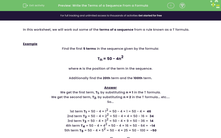# Write the Terms of a Sequence From a Formula

In this worksheet, students will find the required terms of a sequence from a given T formulaKey stage:  KS 3

Curriculum topic:   Algebra

Curriculum subtopic:   Generate Terms of a Sequence

Difficulty level:#### Worksheet Overview

In this activity, we will work out some of the terms of a sequence from a rule known as a T formula.

Example

Find the first 5 terms in the sequence given by the formula:

Tn = 50 - 4n2

where n is the position of the term in the sequence.

Additionally find the 20th term and the 100th term.

We get the first term, T1, by substituting n = 1 into the T formula.

We get the second term, T2, by substituting n = 2 into the T formula... etc....

So...

1st term T1 = 50 - 4 × 12 = 50 - 4 × 1 = 50 - 4 =  46

2nd term T2 = 50 - 4 × 22 = 50 - 4 × 4 = 50 - 16 =  34

3rd term T3 = 50 - 4 × 32 = 50 - 4 × 9 = 50 - 36 =  14

4th term T4 = 50 - 4 × 42 = 50 - 4 × 16 = 50 - 64 =  -14

5th term T5 = 50 - 4 × 52 = 50 - 4 × 25 = 50 - 100 =  -50

Now we find the 20th and 100th terms.

T20 = 50 - 4 × 202 = 50 - 4 × 400 = 50 - 1,600 =  -1,550

T100 = 50 - 4 × 1002 = 50 - 4 × 10,000 = 50 - 40,000 =  -39,950

Let's practise this in the following questions.

### What is EdPlace?

We're your National Curriculum aligned online education content provider helping each child succeed in English, maths and science from year 1 to GCSE. With an EdPlace account you’ll be able to track and measure progress, helping each child achieve their best. We build confidence and attainment by personalising each child’s learning at a level that suits them.

Get started